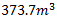# Significant Figures for NEET, JEE and Other Physics Related Exams

Significant Figures for NEET, JEE and other Physics related Exams

Significant Figure - significant means ' something which has meaning or importance'. So Significant numbers are those numbers which are considered important and reliable.

In science we have to measures values of many physical quantities. In those measured values we have to take only significant figures to make the data simple and reliable.

Further we are going to represents some Rules that will help to write the significant figure in any type of values

RULE 1 - All the numbers other than zero are considered to be significant.

For Example - 987 (It has three significant figures)

2468 (It has Four Significant figures)

RULE 2 - If zeroes comes between the two non-zeroes numbers, it will be considered significant.

For Example - 402 (It has three Significant figures)

1001 (It has Four Significant figures)

101.01 (It has Five Significant figures)

RULE 3 - It there is a zero before the decimal, all the zeroes which are comes before the non zero digit are non-significant.

For Example - 0.007 (It has only the Significant figures)

0.0123 (It has only three Significant figures)

0.1457 (It has only four significant figures)

RULE 4 - All the zeroes comes after non-zero digit without any decimal in the number, are considered non significant.

For Example - 14300 (It has three significant figures)

12000 (It has two significant figures)

7000 (It has only one Significant figure)

RULE 5 - All the zeroes comes after non-zero number with decimal point are Significant.

For Example - 1.200 (It has four significant figures)

4.1400 (It has Five Significant Figures)

Rules to write Significant figures in the case of Arithmetic Operations

1. Rule for Addition or Subtraction

In the case of addition and subtraction, the number of significant figures in which the number comes after the operation is the least number in the measurement.

For Example - 1.5 +2.34+1.268 = 5.108 ( The Answer of this measurement in the form of significant figure is 5.1 )

2. Rule for Multiplication and Division

In the case of Multiplication and Division, the number of significant figures in which the number comes after the operation is the least number in the measurement.

For Example - Mass = 2.124g and volume = 1.2 cm3

Density = Mass/Volume = 2.124/1.2 = 1.77gcm-3 ( The Answer of this measurement in the form of significant figure is 1.7gcm-3 )

Rounding Off

RULE 1 - If the uncertain number is smaller than 5 then the last number of significant figure will be same.

For Example - 2.3432 (If this number rounded off upto three terms then the number must be 2.34 because the fourth number is less than 5 so the third term remain same in this case)

RULE 2  - If the uncertain number is greater than 5 then the last number of significant figure will be raised by 1.

For Example - 2.3472 (If this number rounded off upto three terms then the number must be 2.35 because the fourth number is greater than 5 so the third term raised by 1 in this case)

In the case, when dropping number is 5 then the Rule is different for Even and Odd numbers -

RULE 3 - When dropping number is 5 and the previous number is even then the previous number remain same. For Example - 4.325 (Rounding off this number upto three significant figures is 4.32)

RULE 4 - When dropping number is 5 and the previous number is odd then the previous number raised by 1. For Example - 4.335 (Rounding off this number upto three significant figures is 4.34)

NCERT Problems on Significant Figures -

1. Each side of a cube is measured to be 7.203 m. What are the total surface area and the volume of the cube to appropriate significant figures ?

Solution - Given length of a cube = 7.203 ( So answer should be in 4 significant figures )

Volume in significant figure =2. 5.74 g of a substance occupies 1.2 cm3. Express its density by keeping the significant figures in view.

Solution - The minimum numbers of significant figures from both mass and volume are 2, So the calculated answer should be rounded off to 2 significant figures.

3. The number of significant figures in 0.06900 is
Solution - Number of significant figures = 4

4. The sum of the numbers 436.32, 227.2 and 0.301 in appropriate significant figures is
Solution- In the above problem, from all the given values the least significant figures are 3, so the answer should be in 3 figures.
=  436.32+227.2+0.301
= 663.821
= 664
5. The numbers 2.745 and 2.735 on rounding off to 3 significant figures will give
Solution - 2.74 in both cases

Privious Year Questions (PYQs) of NEET and other Exams on Significant Figures -

1. Taking into account of the significant figures, what is the value of 9.99m - 0.0099m ?
(NEET Sep 2020)
Solution - 9.99-0.0099 = 9.9801m
Here we have two digits after the decimal, so the answer will be 9.98

Solution - 5,1 and 2

Practice Problems on Significant Figures -

1. How many significant figure are there in the following values

1. 0.1
2. 0.001
3. 2.017
4. 0.1492
5. 14.001
6. 1.01
7. 0.010
8. 0.007
9. 10.001
10. 100.00
2. Add 0.1, 0.01 and 1.001. Give answer in appropriate significant figures.
3. Subtract 2.42 from 2.847 and give answer in appropriate significant figures.
4. Multiply 0.7 and 0.010 and give answer in appropriate significant figures.
5. Divide 8.448 by 0.4 and give answer in appropriate significant figures.
6. Give answer in appropriate significant figures by squaring 0.24.
7. Give answer in appropriate significant figures by cubing 0.8.
8. Side of the square is 0.40. Find its perimeter in appropriate significant figures.